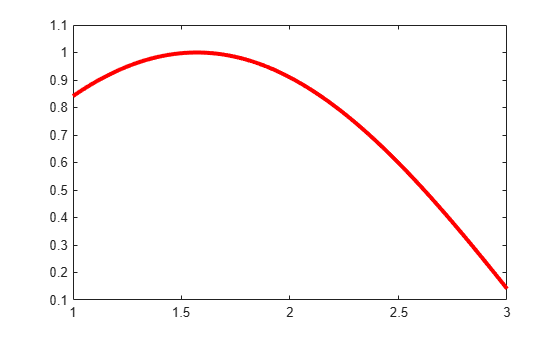Main Content

# fnplt

## Syntax

``fnplt(f)``
``fnplt(f,symbol,interv,linewidth,jumps) ``
``points = fnplt(f,...) ``
``[points, t] = fnplt(f,...) ``

## Description

````fnplt(f)` plots the function in `f` on its basic interval.If f is univariate, then: If f is scalar-valued, `fnplt` plots the graph of f.If f is 2-vector-valued, `fnplt` plots the planar curve.If f is d-vector-valued with d > 2, `fnplt` plots the space curve given by the first three components of f.If f is bivariate, then: If f is scalar-valued, `fnplt` plots the graph of f, using `surf`.If f is 2-vector-valued, `fnplt` plots the image in the plane of a regular grid in its domain.If f is d-vector-valued with d > 2, `fnplt` plots the parametric surface given by the first three components of its values, using `surf`.If f is a function of more than two variables, then `fnplt` plots the bivariate function, obtained by choosing the midpoint of the basic interval in each of the variables other than the first two. NoteThe basic interval for f in B-form is the interval containing all the knots. This means that f is sure to vanish at the endpoints of the basic interval unless the first and the last knot are both of full multiplicity k, with k the order of the spline f. Failure to have such full multiplicity is particularly annoying when f is a spline curve, since the plot of that curve as produced by `fnplt` is then bound to start and finish at the origin, regardless of what the curve might otherwise do.Further, since B-splines are zero outside their support, any function in B-form is zero outside the basic interval of its form. This is very much in contrast to a function in ppform whose values outside the basic interval of the form are given by the extension of its leftmost, respectively rightmost, polynomial piece. ```

example

````fnplt(f,symbol,interv,linewidth,jumps) ` permits you to modify the plotting by the specification of additional input arguments. You can place these arguments in whatever order you like, from the following list:A character vector that specifies a plotting symbol, such as `'-.'` or `'*'`; the default is `'-'`.A scalar to specify the linewidth; the default value is `1`.A character vector that starts with the letter `'j'` to indicate that any jump in the univariate function being plotted appears as a jump. The default is to fill in any jump by a (near-)vertical line.A vector of the form `[a,b]`, to indicate the interval over which to plot the `univariate` function in `f`. If the function in `f` is m-variate, then this optional argument must be a cell array whose ith entry specifies the interval over which the ith argument is to vary. In effect, for this `arg`, the command `fnplt(f,arg,...)` has the same effect as the command `fnplt(fnbrk(f,arg),...)`. The default is the basic interval of `f`.An empty matrix or character vector, to indicate use of default(s). This option is useful when your particular choice depends on some other variables.```
````points = fnplt(f,...) ` plots nothing and returns the two-dimensional points or three-dimensional points it would have plotted instead.```
````[points, t] = fnplt(f,...) ` also returns, for a vector-valued `f`, the corresponding vector `t` of parameter values.```

## Examples

collapse all

This simple example shows how to plot a spline using the `fnplt` function.

Create a vector of data sites.

`x=linspace(0,2*pi,21);`

Generate a spline with the data sites `x` previously created.

`f = spapi(4,x,sin(x))`
```f = struct with fields: form: 'B-' knots: [1x25 double] coefs: [1x21 double] number: 21 order: 4 dim: 1 ```

Finally plot the spline using the `fnplt` function.

`fnplt(f,'r',3,[1 3])`## Input Arguments

collapse all

Function you want to plot, specified as a scalar, vector, ND-array, or a spline in either ppform, B-form or stform.

Symbol used to plot the function, specified as a character vector.

Interval over which to plot the `univariate` function in `f`, specified as a vector. If the function in `f` is m-variate, then this parameter must be a cell array whose i-th entry specifies the interval over which the i-th argument is to vary.

Data Types: `single` | `double`

Width of the plotting line, specified as a scalar.

Data Types: `single` | `double`

Specify how to plot a jump in the univariate function, specified as a character vector. The default is to fill in any jump by a (near-)vertical line.

## Output Arguments

collapse all

Two dimensional or three dimensional points of the function that would have been plotted, returned as a vector or matrix.

Corresponding parameter values of function `f`, returned as a vector or matrix.

## Algorithms

The `fnplt` functions generates a vector `x` of evaluation points by the union of:

1. 101 equally spaced sites filling out the plotting interval

2. Any breakpoints in the plotting interval.

Then `fnplt` evaluates the univariate function f described by `f` at these `x` evaluation points. If f is real-valued, it plots the points (x,f(x)). If f is vector-valued, it plots the first two or three components of f(x).

The bivariate function f described by `f` is evaluated on a 51-by-51 uniform grid if f is scalar-valued or d-vector-valued with d > 2 and the result plotted by `surf`. In the contrary case, f is evaluated along the meshlines of a 11-by-11 grid, and the resulting planar curves are plotted.

## See Also

### Topics

Introduced before R2006a

## Support

#### Machine Learning Challenges: Choosing the Best Classification Model and Avoiding Overfitting

Download white paper